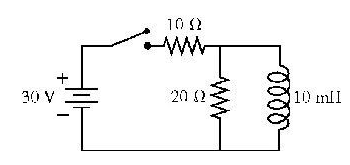# Problem: The switch in the figure has been open for a long time. It is closed at t = 0 s.What is the current through the 20 ohm resistor immediately after the switch is closed?

###### FREE Expert Solution

For resistors in series:

Ohm's law:

$\overline{){\mathbf{V}}{\mathbf{=}}{\mathbf{i}}{\mathbf{R}}}$

The inductor acts as a path of infinite resistance (open circuit) immediately after the switch is closed.###### Problem Details

The switch in the figure has been open for a long time. It is closed at t = 0 s.What is the current through the 20 ohm resistor immediately after the switch is closed?